>

# NCERT Solutions for Class 10 Maths Ch 7 Coordinate Geometry

Chapter 7 Coordinate Geometry NCERT Solutions for Class 10 Maths is available here which will be vey helpful during the preparation of examinations. It will be also useful in completing your homework and understanding concepts clearly. Class 10 Maths NCERT Solutions for Chapter 7 Coordinate Geometry is very essential for scoring good marks in the class test or board examinations.Page No: 161

Exercise 7.1

1. Find the distance between the following pairs of points:
(i) (2, 3), (4, 1) (ii) (−5, 7), (−1, 3) (iii) (a, b), (− a, − b)

(i) Distance between the points is given by(ii) Distance between (−5, 7) and (−1, 3) is given by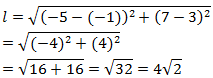(iii) Distance between (ab) and (− a, − b) is given by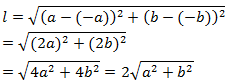2. Find the distance between the points (0, 0) and (36, 15). Can you now find the distance between the two towns A and B discussed in Section 7.2.

Distance between points (0, 0) and (36, 15)Yes, Assume town A at origin point (0, 0).
Therefore, town B will be at point (36, 15) with respect to town A.
And hence, as calculated above, the distance between town A and B will be 39 km.

3. Determine if the points (1, 5), (2, 3) and (-2, -11) are collinear.

Let the points (1, 5), (2, 3), and (- 2,-11) be representing the vertices A, B, and C of the given triangle respectively.Let A = (1, 5), B = (2, 3) and C = (- 2,-11)Since AB + BC ≠ CA
Therefore, the points (1, 5), (2, 3), and ( - 2, - 11) are not collinear.

4. Check whether (5, - 2), (6, 4) and (7, - 2) are the vertices of an isosceles triangle.

Let the points (5, - 2), (6, 4), and (7, - 2) are representing the vertices A, B, and C of the given triangle respectively.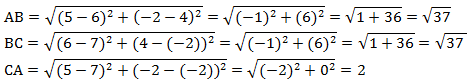Therefore, AB = BC
As two sides are equal in length, therefore, ABC is an isosceles triangle.

5. In a classroom, 4 friends are seated at the points A, B, C and D as shown in the following figure. Champa and Chameli walk into the class and after observing for a few minutes Champa asks Chameli, “Don't you think ABCD is a square?” Chameli disagrees.
Using distance formula, find which of them is correct.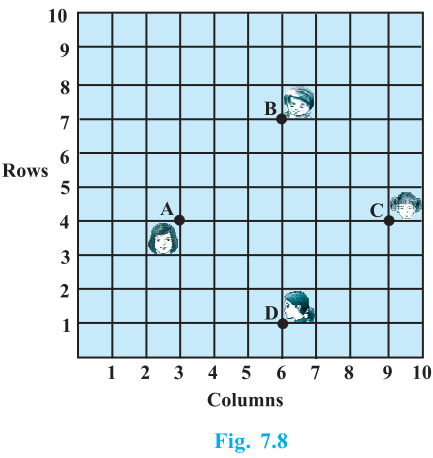Clearly from the figure, the coordinates of points A, B, C and D are (3, 4), (6, 7), (9, 4) and (6, 1).
By using distance formula, we get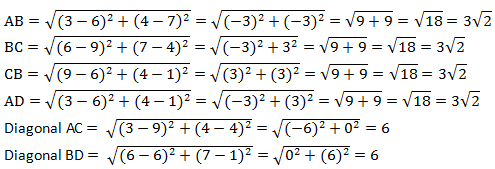It can be observed that all sides of this quadrilateral ABCD are of the same length and also the diagonals are of the same length.
Therefore, ABCD is a square and hence, Champa was correct

6. Name the type of quadrilateral formed, if any, by the following points, and give reasons for your answer:
(i) (- 1, - 2), (1, 0), (- 1, 2), (- 3, 0)
(ii) (- 3, 5), (3, 1), (0, 3), (- 1, - 4)
(iii) (4, 5), (7, 6), (4, 3), (1, 2)

Let the points ( - 1, - 2), (1, 0), ( - 1, 2), and ( - 3, 0) be representing the vertices A, B, C, and D of the given quadrilateral respectively.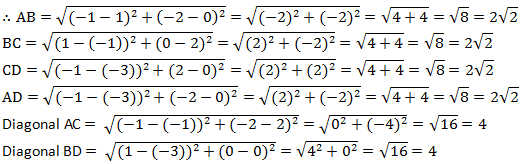It can be observed that all sides of this quadrilateral are of the same length and also, the diagonals are of the same length. Therefore, the given points are the vertices of a square.

(ii) Let the points ( - 3, 5), (3, 1), (0, 3), and ( - 1, - 4) be representing the vertices A, B, C, and D of the given quadrilateral respectively.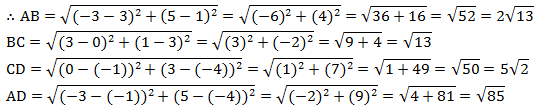It can be observed that all sides of this quadrilateral are of different lengths. Therefore, it can be said that it is only a general quadrilateral, and not specific such as square, rectangle, etc.

(iii) Let the points (4, 5), (7, 6), (4, 3), and (1, 2) be representing the vertices A, B, C, and D of the given quadrilateral respectively.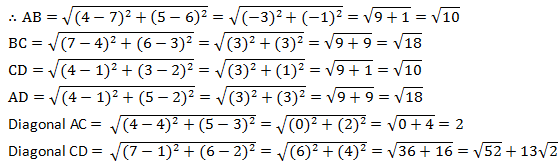It can be observed that opposite sides of this quadrilateral are of the same length. However, the diagonals are of different lengths. Therefore, the given points are the vertices of a parallelogram.

7. Find the point on the x-axis which is equidistant from (2, - 5) and (- 2, 9).

We have to find a point on x-axis. Therefore, its y-coordinate will be 0.
Let the point on x-axis be (x,0)(- 2)2 + 25 = (x - 2)2 + 81
x2 + 4 - 4x + 25 = x2 + 4 + 4x + 81
8x = 25 -81
8x = -56
x = -7
Therefore, the point is ( - 7, 0).

8. Find the values of y for which the distance between the points P (2, - 3) and Q (10, y) is 10 units.

It is given that the distance between (2, - 3) and (10, y) is 10.64 + (y + 3)2 = 100
(y +3)2 = 36
y + 3 = ±6
y + 3 = +6 or y + 3 = -6
Therefore, y = 3 or -9

9. If Q (0, 1) is equidistant from P (5, - 3) and R (x, 6), find the values of x. Also find the distance QR and PR.

PQ = QR41 = x2 + 25
16 = x2
x = ±4
Therefore, point R is (4, 6) or ( - 4, 6).
When point R is (4, 6),10. Find a relation between x and y such that the point (x, y) is equidistant from the point (3, 6) and (- 3, 4).

Point (x, y) is equidistant from (3, 6) and ( - 3, 4).x2 + 9 - 6x + y2 + 36 - 12y = x2 + 9 + 6x + y2 + 16 - 8y
36 - 16 = 6x + 6x + 12y - 8y
20 = 12x + 4y
3x + y = 5
3x + y - 5 = 0

Exercise 7.2

1. Find the coordinates of the point which divides the join of (- 1, 7) and (4, - 3) in the ratio 2:3.

Let P(x, y) be the required point. Using the section formula, we get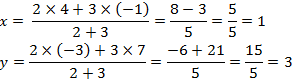Therefore, the point is (1, 3).

2. Find the coordinates of the points of trisection of the line segment joining (4, -1) and (-2, -3).Let P (x1, y1) and Q (x2, y2) are the points of trisection of the line segment joining the given points i.e., AP = PQ = QB
Therefore, point P divides AB internally in the ratio 1:2.3. To conduct Sports Day activities, in your rectangular shaped school ground ABCD, lines have been drawn with chalk powder at a distance of 1 m each. 100 flower pots have been placed at a distance of 1 m from each other along AD, as shown in the following figure. Niharika runs 1/4th the distance AD on the 2nd line and posts a green flag. Preet runs 1/5th the distance AD on the eighth line and posts a red flag. What is the distance between both the flags? If Rashmi has to post a blue flagexactly halfway between the line segment joining the two flags, where should she post her flag?It can be observed that Niharika posted the green flag at 1/4th of the distance AD i.e., (1×100/4)m = 25m from the starting point of 2nd line. Therefore, the coordinates of this point G is (2, 25).
Similarly, Preet posted red flag at 1/5 of the distance AD i.e., (1×100/5) m = 20m from the starting point of 8th line. Therefore, the coordinates of this point R are (8, 20).
Distance between these flags by using distance formula = GRTherefore, Rashmi should post her blue flag at 22.5m on 5th line.

4. Find the ratio in which the line segment joining the points (-3, 10) and (6, - 8) is divided by (-1, 6).

Let the ratio in which the line segment joining ( -3, 10) and (6, -8) is divided by point ( -1, 6) be k:1.
Therefore, -1 = 6k-3/k+1
-k - 1 = 6k -3
7k = 2
k = 2/7
Therefore, the required ratio is 2:7.

5. Find the ratio in which the line segment joining A (1, - 5) and B (- 4, 5) is divided by the x-axis. Also find the coordinates of the point of division.

Let the ratio in which the line segment joining A (1, - 5) and B ( - 4, 5) is divided by x-axis be k:1.
Therefore, the coordinates of the point of division is (-4k+1/k+1, 5k-5/k+1).
We know that y-coordinate of any point on x-axis is 0.
∴ 5k-5/k+1 = 0
Therefore, x-axis divides it in the ratio 1:1.6. If (1, 2), (4, y), (x, 6) and (3, 5) are the vertices of a parallelogram taken in order, find x and y.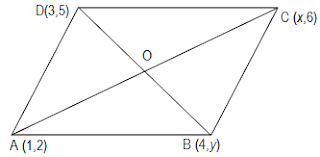Let A,B,C and D be the points (1,2) (4,y), (x,6) and (3,5) respectively.
Mid point of diagonal AC isand Mid point of Diagonal BD isSince the diagonals of a parallelogram bisect each other, the mid point of AC and BD are same.
x+1/2 = 7/2 and 4 = 5+y/2
x + 1 = 7 and 5 + y = 8
x = 6 and y = 3

7. Find the coordinates of a point A, where AB is the diameter of circle whose centre is (2, - 3) and B is (1, 4).

Let the coordinates of point A be (x, y).
Mid-point of AB is (2, - 3), which is the center of the circle.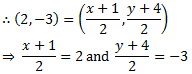⇒ x + 1 = 4 and y + 4 = -6
⇒ x = 3 and y = -10
Therefore, the coordinates of A are (3,-10).

8. If A and B are (–2, –2) and (2, –4), respectively, find the coordinates of P such that AP = 3/7 AB and P lies on the line segment AB.The coordinates of point A and B are (-2,-2) and (2,-4) respectively.
Since AP = 3/7 AB
Therefore, AP:PB = 3:4
Point P divides the line segment AB in the ratio 3:4.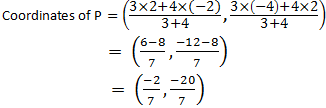9. Find the coordinates of the points which divide the line segment joining A (- 2, 2) and B (2, 8) into four equal parts.From the figure, it can be observed that points X, Y, Z are dividing the line segment in a ratio 1:3, 1:1, 3:1 respectively.10. Find the area of a rhombus if its vertices are (3, 0), (4, 5), (-1, 4) and (-2,-1) taken in order. [Hint: Area of a rhombus = 1/2(product of its diagonals)]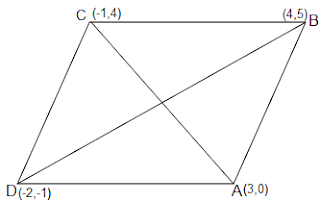Let (3, 0), (4, 5), ( - 1, 4) and ( - 2, - 1) are the vertices A, B, C, D of a rhombus ABCD.Page No: 170

Exercises 7.3

1. Find the area of the triangle whose vertices are:
(i) (2, 3), (-1, 0), (2, -4)
(ii) (-5, -1), (3, -5), (5, 2)

(i) Area of a triangle is given by
Area of triangle = 1/2 {x1 (y2y3)+ x2 (y3y1)+ x3 (y1y2)}
Area of the given triangle = 1/2 [2 { 0- (-4)} + (-1) {(-4) - (3)} + 2 (3 - 0)]
= 1/2 {8 + 7 + 6}
= 21/2 square units.

(ii) Area of the given triangle = 1/2 [-5 { (-5)- (4)} + 3(2-(-1)) + 5{-1 - (-5)}]
= 1/2{35 + 9 + 20}
= 32 square units

2. In each of the following find the value of 'k', for which the points are collinear.
(i) (7, -2), (5, 1), (3, -k
(ii) (8, 1), (k, -4), (2, -5)

(i) For collinear points, area of triangle formed by them is zero.
Therefore, for points (7, -2) (5, 1), and (3, k), area = 0
1/2 [7 { 1- k} + 5(k-(-2)) + 3{(-2) + 1}] = 0
7 - 7k + 5k +10 -9 = 0
-2k + 8 = 0
k = 4

(ii) For collinear points, area of triangle formed by them is zero.
Therefore, for points (8, 1), (k, - 4), and (2, - 5), area = 0
1/2 [8 { -4- (-5)} + k{(-5)-(1)} + 2{1 -(-4)}] = 0
8 - 6k + 10 = 0
6k = 18
k = 3

3. Find the area of the triangle formed by joining the mid-points of the sides of the triangle whose vertices are (0, -1), (2, 1) and (0, 3). Find the ratio of this area to the area of the given triangle.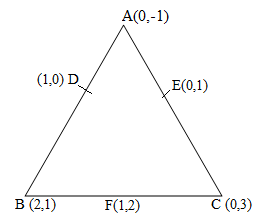Let the vertices of the triangle be A (0, -1), B (2, 1), C (0, 3).
Let D, E, F be the mid-points of the sides of this triangle. Coordinates of D, E, and F are given by
D = (0+2/2 , -1+1/2) = (1,0)
E = (0+0/2 , -3-1/2) = (0,1)
F = (2+0/2 , 1+3/2) = (1,2)
Area of a triangle = 1/2 {x1 (y2 - y3) + x2 (y3 - y1) + x3 (y1 - y2)}
Area of ΔDEF = 1/2 {1(2-1) + 1(1-0) + 0(0-2)}
= 1/2 (1+1) = 1 square units
Area of ΔABC = 1/2 [0(1-3) + 2{3-(-1)} + 0(-1-1)]
= 1/2 {8} = 4 square units
Therefore, the required ratio is 1:4.

4. Find the area of the quadrilateral whose vertices, taken in order, are (-4, -2), (-3, -5), (3, -2) and (2, 3).Let the vertices of the quadrilateral be A ( - 4, - 2), B ( - 3, - 5), C (3, - 2), and D (2, 3). Join AC to form two triangles ΔABC and ΔACD.
Area of a triangle = 1/2 {x1 (y2 - y3) + x2 (y3 - y1) + x3 (y1 - y2)}
Area of ΔABC = 1/2 [(-4) {(-5) - (-2)} + (-3) {(-2) - (-2)} + 3 {(-2) - (-5)}]
=  1/2 (12+0+9)
= 21/2 square units
Area of ΔACD = 1/2 [(-4) {(-2) - (3)} + 3{(3) - (-2)} + 2 {(-2) - (-2)}]
= 1/2 (20+15+0)
= 35/2 square units
Area of ☐ABCD  = Area of ΔABC + Area of ΔACD
= (21/2 + 35/2) square units = 28 square units

5. You have studied in Class IX that a median of a triangle divides it into two triangles of equal areas. Verify this result for ΔABC whose vertices are A (4, - 6), B (3, - 2) and C (5, 2).Let the vertices of the triangle be A (4, -6), B (3, -2), and C (5, 2).
Let D be the mid-point of side BC of ΔABC. Therefore, AD is the median in ΔABC.
Coordinates of point D = (3+5/2, -2+2/2) = (4,0)
Area of a triangle = 1/2 {x1 (y2 - y3) + x2 (y3 - y1) + x3 (y1 - y2)}
Area of ΔABD = 1/2 [(4) {(-2) - (0)} + 3{(0) - (-6)} + (4) {(-6) - (-2)}]
= 1/2 (-8+18-16)
= -3 square units
However, area cannot be negative. Therefore, area of ΔABD is 3 square units.
Area of ΔABD = 1/2 [(4) {0 - (2)} + 4{(2) - (-6)} + (5) {(-6) - (0)}]
= 1/2 (-8+32-30)
= -3 square units
However, area cannot be negative. Therefore, area of ΔABD is 3 square units.
The area of both sides is same. Thus, median AD has divided ΔABC in two triangles of equal areas.

### NCERT Solutions for Class 10 Maths Chapter 7 Coordinate Geometry

Chapter 7 Class 10 Maths NCERT Solutions is given here which will help you in revising the chapter properly and knowing application formulae. The chapter is divided into 4 topics that will improve your knowledge regarding Coordinate Geometry.

• Introduction: Coordinate geometry is an algebraic tool for studying geometry of figures. In earlier class, we studied how to locate the position of a point on a plane and various terms related to it.

• Distance Formula: You will learn how to find the distance between the two points whose coordinates are given.

• Area of the Triangle: You will learn to find the area of the triangle formed by three given points.

• Section Formula: You will study how to find the coordinates of the point which divides a line segment joining two given points in a given ratio.

You can also access other study resources prepared by Studyrankers experts:

There are total 4 exercises in the NCERT Solutions for Class 10 Maths Chapter 7 Coordinate Geometry which are very helpful in increasing concentration among students. If you want exercisewise NCERT Solutions then you can get them below.

NCERT Solutions for Class 10 Maths Chapters:

 Chapter 1 Real Numbers Chapter 2 Polynomials Chapter 3 Pair of Linear Equations in Two Variables Chapter 4 Quadratic Equations Chapter 5 Arithmetic Progressions Chapter 6 Triangles Chapter 8 Introduction To Trigonometry Chapter 9 Some Applications Of Trigonometry Chapter 10 Circles Chapter 11 Constructions Chapter 12 Areas Related To Circles Chapter 13 Surface Areas And Volumes Chapter 14 Statistics Chapter 15 Probability

FAQ on Chapter 7 Coordinate Geometry

#### How many exercises in Chapter 7 Coordinate Geometry

There are only 4 exercises in the Chapter 7 Class 10 Maths Coordinate Geometry in which last one is optional. These answers are prepared by experts of Studyrankers who have vast experience in teaching. These will help the students  in learning the concepts efficiently.

#### What is Coordinate Geometry?

Coordinate Geometry is the branch of Mathematics in which algebraic methods are used to solve geometrical problems is known as coordinate geometry.

#### Find the point on y-axis which is equidistant from the points (5, − 2) and (− 3, 2).

Let the required point be P (0, y) The given points are A (5, − 2) and B (− 3, 2)
∴ PA = PB
⇒ PA2 = PB2
∴(5 − 0)2 + (− 2 − y)2 =(− 3 − 0)2 + (2 − y)2
⇒ 52 + (− 2 − y)2 = (− 3)2 + (2 − y)2
⇒ 25 + 4 + y2 + 4y = 9 + 4 + y2 − 4y
⇒ 25 + 4y = 9 − 4y
⇒ 8 y = − 16 ⇒ y = − 2

Thus, the required point is (0, − 2)

#### If the points A (4, 3) and B (x, 5) are on the circle with the centre O (2, 3), find the value of x.

Let O (2, 3) be the centre of the circle.
∴ OA = OB ⇒ OA2 = OB2
⇒ (4 − 2)2 + (3 − 3)2 =(x − 2)2 + (5 − 3)2
⇒ 22 =(x − 2)2 + 22
⇒ (x − 2)2 = 0
⇒ x −2 = 0
⇒ x = 2

Thus, the required value of x is 2.